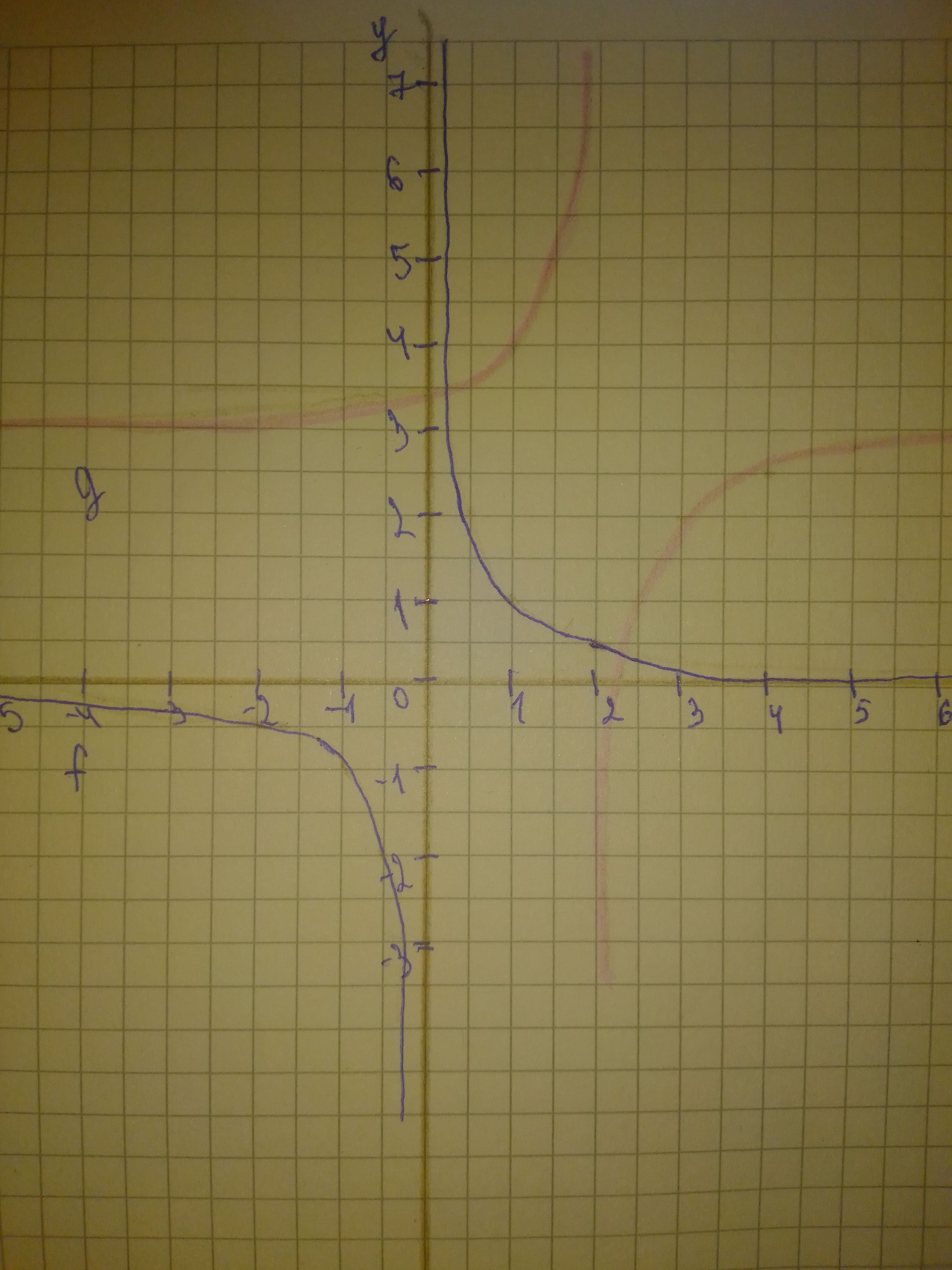midtlinjeg

2021-01-31

Use long division to rewrite the equation for g in the form
$\text{quotient}+\frac{remainder}{divisor}$
Then use this form of the function's equation and transformations of
$f\left(x\right)=\frac{1}{x}$
to graph g.
$g\left(x\right)=\frac{3x-7}{x-2}$wheezym

Step 1
$g\left(x\right)=\frac{3x-7}{x-2}$

The quotient is 3 and the remainer is $\frac{-1}{x-2}$
$g\left(x\right)=3-\frac{1}{x-2}$
We can graph g by horizontal shifting f 2 units right then vertical shifting 3 units up and reflect about y-axis.
Step 2........................

Do you have a similar question?